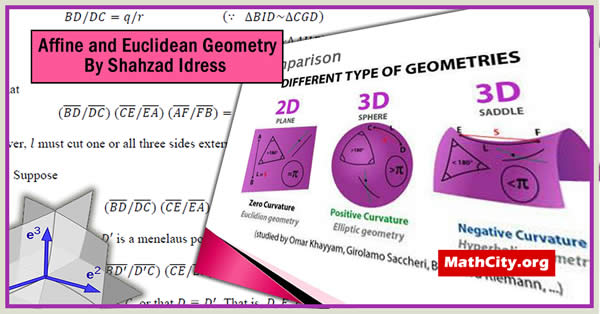# Affine and Euclidean Geometry by Shahzad IdressThese notes are sent by shahzad-idress. We acknowledged his efforts to published these notes on MathCity.org.

These are short notes containing topics related to Affine and Euclidean Geometry. The main sections includes “Vector Space and Affine Geometry”, “Euclidean Geometry”, “Orthogonal Transformations” and “Platonic Polyhedra”.

 Name Affine and Euclidean Geometry Shahzad Idress 41 pages PDF (see Software section for PDF Reader) 1.44 mB
• Linear & Affine Subspaces
• Linear Spaces & Subspaces
• Affine Subspaces: Affine Combination, Convex Combination, Dimension of an Affine Subspace, Affine Span, Affine Independence, Affine Basis, Barycentric or Affine Coordinates, Hyperplane in $R^n$
• Inner Product and Euclidean Geometry
• Inner Product Spaces: Inner Product, Euclidean Inner Product, Norm of a Vector, Distance Between Two Points, Cauchy-Schwarz Inequality, Angle Between Two Vectors.
• The Laws of Cosine and Sines: The Law of Cosine, Pythagoras Theorem, Parallelogram Law, The Law of Sines,
• Euclidean Constructions: The Euclidean Tools, The Method of Loci
• Classical Theorems in Affine Geometry
• Sensed Magnitudes, Positive and Negative Segments, Range of Points and Complete Range, Basic Theorems.
• Menelaus, Ceva and Desargues Theorems: The ratio 𝑨𝑷/𝑷𝑩, Angles Associated with Parallel Lines, Thales’ Theorem, Menelaus Point, Menelaus’ Theorem, Cevian Line, Ceva’s Theorem, Copolar Triangles, Coaxial Triangles, Desargues’ Theorem.
• Platonic Polyhedra
• Platonic Solids, Convex Set, Convex Polyhedron, Regular Polyhedron, Classification of Platonic Solids, Euler’s Formula, Duality.